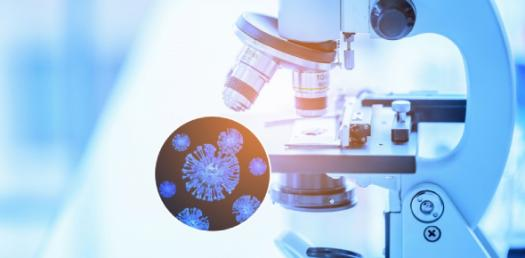# Science Rapid Quiz 1

10 Questions | Total Attempts: 85SettingsCreate your own QuizThis quiz will be conducted every Thursday

• 1.
Which of the following is a non metal that remains liquid at room temperature?
• A.

Phosphorous

• B.

Bromine

• C.

Chlorine

• D.

Helium

• 2.
A car traveling at 50 km/hr changes its speed to 110 km/hr is 5 seconds. The acceleration of the car during this time is
• A.

5 km/hr/sec

• B.

12 km/hr/sec

• C.

50 km/hr/sec

• D.

60 km/hr/sec

• 3.
_________ is the biggest planet in our solar system.
• 4.
Who was awarded the 1921 Nobel Prize in Physics for his work on theoretical physics?
• A.

Ernst Rutherford

• B.

Elbert Einstien

• C.

Charles Hard Townes

• D.

Rosalyn Sussman Yalow

• 5.
A vector quantity has
• A.

Magnitude only

• B.

Direction only

• C.

Both magnitude and direction

• D.

Neither magnitude or direction

• 6.
DNA is the shortened form of the term ‘Deoxyribonucleic acid’?
• A.

True

• B.

False

• 7.
The sum of all the external forces on a system of particles is zero. Which of the following must be true of the system?
• A.

The total mechanical energy is constant.

• B.

The total potential energy is constant.

• C.

The total kinetic energy is constant.

• D.

The total linear momentum is constant.

• E.

It is in static equilibrium

• 8.
The two elements that are frequently used for making transistors are
• A.

Boron and Aluminium

• B.

Silicon and Germanium

• C.

Iridium and Tungsten

• D.

Niobium and Columbium

• 9.
Reading of a barometer going down is an indication of
• A.

Snow

• B.

Storm

• C.

Intense heat

• D.

Rainfall

• 10.
Which of the following is a non metal that remains liquid at room temperature?
• A.

Phosphorous

• B.

Bromine

• C.

Chlorine

• D.

Helium

Related TopicsBack to top
×

Wait!
Here's an interesting quiz for you.